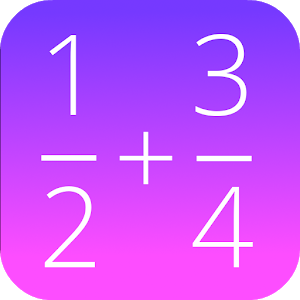English

Every day we offer FREE licensed Android apps and games you’d have to buy otherwise.\$0.99
EXPIRED

# Android Giveaway of the Day - Fractions Math Pro

This math app is a fractions calculator with various functions!
\$0.99 EXPIRED
User rating: 4 (100%) 0 (0%)

This giveaway offer has expired. Fractions Math Pro is now available on the regular basis.

This calculator is the pro version of 'Fractions Math', and completely without advertisement.

This math app is a fractions calculator with various functions:

- add, subtract, multiply and divide fractions.
- convert fractions in decimals,
- simplify fractions
- compare fractions
- find the lowest common denominator
- fraction trainer, to learn fraction calculations

GK Apps

Education

4.0

279k

Everyone

1.6 and up

## Comments on Fractions Math Pro

Thank you for voting!